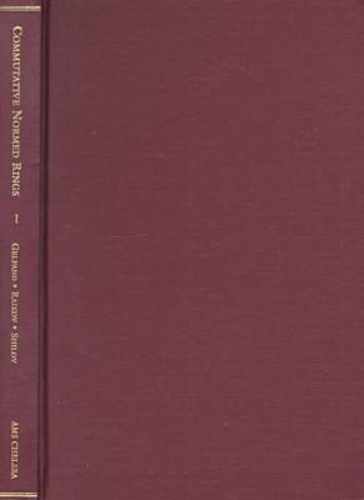•# Commutative Normed Rings - AMS Chelsea Publishing (Hardback)

£49.95
Hardback Published: 30/10/1999
• We can order this

Usually dispatched within 3 weeks

From the Preface (1960): ""This book is devoted to an account of one of the branches of functional analysis, the theory of commutative normed rings, and the principal applications of that theory. It is based on [the authors'] paper written...in 1940, hard on the heels of the initial period of the development of this theory...""The book consists of three parts. Part one, concerned with the theory of commutative normed rings and divided into two chapters; the first containing foundations of the theory and the second dealing with more special problems. Part two deals with applications to harmonic analysis and is divided into three chapters. The first chapter discusses the ring of absolutely integrable functions on a line with convolution as multiplication and finds the maximal ideals of this ring and some of its analogues.In the next chapter, these results are carried over to arbitrary commutative locally compact groups and they are made the foundation of the construction of harmonic analysis and the theory of characters. A new feature here is the construction of an invariant measure on the group of characters and a proof of the inversion formula for Fourier transforms that is not based on theorems on the representation of positive-definite functions or positive functionals...The last chapter of the second part - the most specialized of all the chapters - is devoted to the investigation of the ring of functions of bounded variation on a line with multiplication defined as convolution, including the complete description of the maximal ideals of this ring. The third part of the book is devoted to the discussion of two important classes of rings of functions: regular rings and rings with uniform convergence.The first of the chapters essentially studies the structure of ideals in regular rings. The chapter ends with an example of a ring of functions having closed ideals that cannot be represented as the intersections of maximal ideals. The second chapter discusses the ring \$C(S)\$ of all bounded continuous complex functions on completely regular spaces \$S\$ and various of its subrings...""Since noncommutative normed rings with an involution are important for group-theoretical applications, the paper by I. M. Gelfand and N. A. Naimark, 'Normed Rings with an Involution and their Representations', is reproduced at the end of the book, slightly abridged, in the form of an appendix...This monograph also contains an account of the foundations of the theory of commutative normed rings without, however, touching upon the majority of its analytic applications...""The reader [should] have knowledge of the elements of the theory of normed spaces and of set-theoretical topology. For an understanding of the fourth chapter, [the reader should] also know what a topological group is. It stands to reason that the basic concepts of the theory of measure and of the Lebesgue integral are also assumed to be known...

Publisher: American Mathematical Society
ISBN: 9780821820223
Weight: 567 g
Dimensions: 241 x 159 mm